# Solve Vehicle Routing Problem With Reinforcement Learning

Last updated: Apr 15, 2019

This is a short review of a framework for solving Vehicle Routing Problem (VRP) using deep reinforcement learning.

VRP is relevant to many real-world problems. Imagine a waste collection company has a number of clients with varying amounts of solid waste, waiting at various locations. It wants to design efficient routes for its fleet of trucks such that all clients (their demands) are served collectively by the trucks and the total distance of the routes is minimal. If your problem has a shape like this, well, it is about time we talked about VRP.

What exactly is VRP? In the two previous posts, we already talked about Traveling Salesperson Problem (TSP) in other posts and mentioned that TSP is a special case of VRP. Recall TSP has a single salesperson (vehicle) that wants to visit $n$ cities (a complete, weighted, undirected graph) exactly once most efficiently. In addition, the salesperson starts from one of the cities called a depot and must return to it at the end. As such, TSP is a single route problem.

### $m$-Traveling Salespeople Problem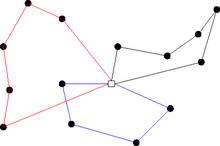A diagram for multi-route problem. Credit: link.

Now, consider your company grew a bit and managed to hire $m(\ge 1)$ salespeople for the same set of cities. How do we plan $m$ distinct routes that cover all cities at least once using a single shared depot? This problem is called $m$-TSP. Interestingly, $m$-TSP is, at most (in worst time complexity), as difficult as 1-TSP. This follows from the observation that $m$-TSP can be re-formulated to 1-TSP at no significant cost. The idea is to make $m$ copies of the shared depot $dp_1, dp_2, \dots, dp_m$ and insert them into an input graph as if they are distinct $\{x_1,\dots,x_n, dp_1, \dots, dp_m\}$. Next, we assign an infinite weight between any made-up $m$ vertices, so no edge incident to any pair of them would be used. Of course, as for the edges incident to $n$ vertices, we just copy the weights of the original depot. The problem has been reduced to 1-TSP of $(n + m)$ cities. The reduction took $\Theta(n \cdot m)$. Once we solve the 1-TSP (not easy), the solution will be of the form $\pi = (d\rightarrow \bar{x}_1 \rightarrow d \rightarrow \bar{x}_2 \rightarrow \dots \rightarrow d)$ where $\bar{x}$ is a subset of the original vertices $x$ (not $dp$). This is because the solution must visit every depot only once and between any two depots, there will be non-empty $\bar{x}$ (because, no edge connecting two depots will be used). Then, we decompose $\pi$ into m tours $\pi_1 = (dp, \bar{x}_1, dp), \dots, \pi_m = (dp, \bar{x}_m, dp)$. Assuming $\pi$ is optimal, how do we know if the m tours $\pi_1, \dots, \pi_m$ are optimal? We can prove by contradiction: assume our $m$ tours are not optimal. This implies there exists at least one inefficient route (different from the optimal). Since $\pi$ is optimal, every sub-path of $\pi$ should also be optimal. Hence, a contradiction.

### Vehicle Routing Problem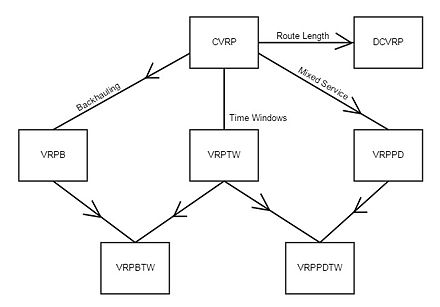A map of VRP variants. Credit: link.

Now, congratulations on your company’s even bigger success. Your business now expanded to an item pickup business. You have a fleet of $m$ trucks (salespeople) and built multiple storage houses. That is, it may have multiple depots and additional constraints on vehicle capacity, maximum distance per route, and the likes. Then, we have a VRP that is intrinsically more complicated than TSP. Notice in VRP, we sometimes relax the “visit-only-once” constraint; due to other constraints, a feasible solution may not exist if we enforced it. As a general rule of thumb, VRP is tackled in practice almost exclusively by heuristics that has two major schemes1:

1. Cluster first and route next: partition a given area into sub-areas and solve a single route problem for each sub-area.

2. Route first and cluster second: solve a single route problem to produce a single optimal route (likely, local) and split the route into sub-routes.

As expected, solving VRP is NP-hard. Exact methods are not a practical choice, unless the size of a problem is very small. As such, heuristics and meta-heuristics are used. If one can expect to solve a set of problem instances with some invariant pattern, one may design a heuristic that attacks that specific pattern. Such a heuristic, however, may quite overfit the training data and not generalize well across different types of problems in which meta-heuritics can be more robust.

### Single-Depot Vehicle Routing Problem with Deep Reinforcement Learning

Now we talk about the paper of interest. For simplicty, we focus on a single-depot VRP with a single vehicle of a limited capacity. We are given $n$ customers $\{x^1,\dots,x^n\}$ to visit at least once. As opposed to TSP, our input consists of two types of information: static and dynamic: $x_t^i = (s^i, d^i_t)$ that can change over time $t = 0, 1, \dots$. An example of the static information, $s^i$, would be the 2d coordinate of a customer, whereas $d_t^i$ would be the customer’s demand at time $t$. Let $x_t = \{x^1_t, \dots, x^n_t \}$ the input state at time $t$.

Just like TSP, our goal is to plan a tour (a sequence of customers) such that the total cost is minimal. Since we have the notion of demands and other constraints, unlike TSP, a tour must satisfy all customer demands at which time it finishes and additional constraints at all times. Notably, the tour may visit the same customer more than once if the customer’s demand is larger than the vehicle’s capacity (available space at the time of visit), in which case it must return to the depot to unload items and refill its capacity.

The crucial difference from TSP is that an input may change in time, as a function of the vehicle’s trajectory so far. This sounds like a problem RL can solve (as explained in the previous posts) since it naturally forms a sequence of random variables $X_0, Y_0, X_1, Y_1, \dots, Y_{T(X_0)}$ that may lead to simple factorizations. We assume some unknown transition $P(X_{t+1} \vert Y_t, X_t)$ and an unknown initial distribution $P(X_0)$. We make conditional independence assumptions $P(X_{t+1} \vert Y_{t+1}, X_t) = P(X_{t+1} \vert Y_{\le t+1}, X_{\le t})$ and $P(X_{t+1} \vert Y_{t}, X_t) = P(Y_{t+1} \vert Y_{\le t}, X_{\le t})$. This leads to a trajectory distribution under a policy $P(Y_{t+1} \vert Y_t, X_t; W)$.

Now, if we assume a deterministic dynamics $X_{t+1} = f(Y_{t+1}, X_t)$, the formulation can be reduced to $P(\tau \vert X_0) = \mathbb{I}(p(\tau) \ne 0)\prod_{t=0}^T P(Y_{t+1} \vert Y_t, X_t)$ which we aim to represent with our model. In either case, we can apply a model-free RL algorithm such as REINFORCE. If we knew the dynamics $f$, we can basically plan the entire trajectory by internal simulation (open-loop plan). Even if we assume $f$ is unknown, we assume the states $X_{t}, \forall t$ are observable, so we can condition on them (closed-loop plan). We want to train our model $W$ such that the most probable trajectory, as computed by our model, corresponds to the trajectory of the optimal tour.

Now, can we use Pointer Networks (PN) for this problem like we did for TSP? Yes, but probably not a practical idea. Suppose, at $t=0$, PN computes a forward pass up to the first element of a tour $y_1 \sim p(y_1 \vert y_0, x_0; W)$ where $y_0$ is some arbitrary token and $W$ the model parameters. Next suppose, at $t=1$, the first input changes such that $d_0^1 \ne d_1^1$. In this case, PN would need to recompute all hidden states that depend on $x_0^1$. Moreover, accumulating gradients for backpropagation will be hard as we would need to keep track of when and what input elements changed.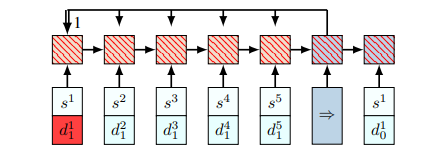Limitation of the Pointer Network. Credit: link.

Now, how can we make the effect of input changes on forward/backward passes as local as possible? The main idea of the paper is quite simple: we stop treating inputs as sequences. We replace the RNN encoder with a set of embeddings $(\bar{x}_t^1, \dots, \bar{x}_t^n)$ where $\bar{x}_t^i = f(x_t^i)\in \mathbb{R}^D$ and $f$ can be some basis feature or in the paper’s case, 1-dimensional convolutional layers (apply $D$ conv. filters to the input matrix $\mathbb{R}^{n \times d}$ to get $\mathbb{R}^{n \times D}$). Notice the filter weights do not depend on $n$ so we are free to vary the input length. Thanks to this input break-up scheme, the effect of input changes is much local (see figure below). Plus, shaving off an RNN may be a good thing any way in the computational perspective.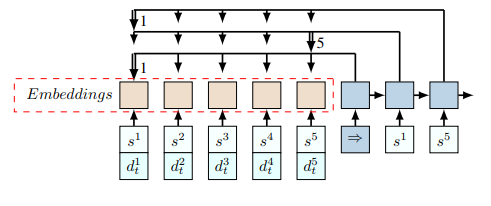An alternative model. Credit: link.

Notice the decoder does not start with the last input encoding $(h_0 \ne \bar{x}_t^n)$ and that it is conditioned only on static inputs to reduce the amounts of recomputation in the RNN decoder. Then, how does the decoder get information about dynamic inputs? Well, at each decoding step, it just needs to look at the latest set of embeddings and apply a context-based attention mechanism. Concretely,

Notice $c_t$ depends on $\bar{x}_t$ (that includes the dynamic elements) even though $h_t$ does not. Just like PN, this model just points to one element (vertex) of the input (graph) (as opposed to chaining the prediction to the next decoder input).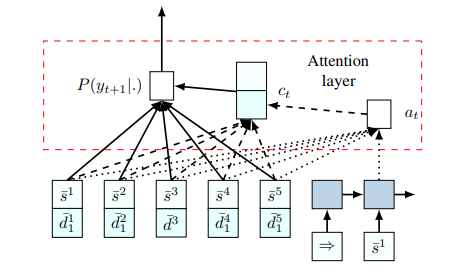The attention mechanism of the alternative model. Credit: link.

Well, that’s all. The rest of the training and inference details are similar to the Neural Combinatorial Optimization paper we talked about earlier.The paper’s experiments feature comparisons to classical method baselines Clarke-Wright and Sweep which it beat. I am not quite sure why they did not include Google OR tools. Also I am not quite sure if an RNN decoder was needed at all as it has a closed-loop control system (i.e. take one action, observe the next state, and so on)–it can still predict the end of a tour. If one were to keep the closed loop syste, it may be a good idea to design an environment that informs the feasibility and optimality of a solution through a well-engineered reward function.

1. http://web.mit.edu/urban_or_book/www/book/chapter6/6.4.9.html